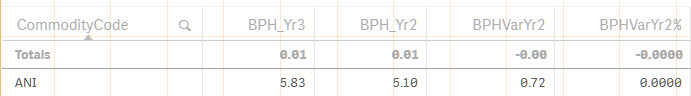Qlik Sense App Development

Highlighted
Contributor II

Problem with variable evaluation

I have variables set up for the following calculations:

I am displaying these variables via Master Measures in a straight table in Qlik Sense 3.1

Variable Name:  vBPHYr2

Definition:

Sum({\$<Year = {"\$(=max({1} Year)-1)"}>} HoursWorked) /

This gets me a "Boxes Per Hour" value that evaluates correctly. For example, I get a value of 5.83 for the selected Product.

Variable Name:  vBPHYr3

Definition:

Sum({\$<Year = {"\$(=max({1} Year)-2)"}>} HoursWorked) /

Evaluates correctly as 5.10 for the specified year and selected Product.

Variable Name: vBPHYr2_Var

Definition:  \$(vBPHYr3) - \$(vBPHYr2)

Evaluates correctly as 0.72

Now I want to take vBPHYr2_Var and divide by vBPHYr3 to get the variance as a percentage.

Variable Name:  vBPHYr2_Var%

Definition:  \$(vBPHYr2_Var) / \$(vBPHYr3)

This evaluates to 0

Thank you.

Tags (3)
1 Solution

Accepted SolutionsMVP

Re: Problem with variable evaluation

This worked

(\$(vBPHYr2_Var))/(\$(vBPHYr3))

8 RepliesMVP

Re: Problem with variable evaluation

0.72/5.10 seems like a small number, do you have the decimals set to 0 may be? Try increasing the number of decimals to see value?

Contributor II

Re: Problem with variable evaluation

I tried setting decimal to four places and still getting zero.MVP

Re: Problem with variable evaluation

Give this a shot

(\$(vBPHYr2_Var))/\$(vBPHYr3)

Contributor II

Re: Problem with variable evaluation

Still zero.MVP

Re: Problem with variable evaluation

Would you be able to share a sample?

Contributor II

Re: Problem with variable evaluation

Here you go. Note:  The sheet called Boxes Per Hour Detail - 3 Years has the straight table I'm referencing for testing.MVP

Re: Problem with variable evaluation

This worked

(\$(vBPHYr2_Var))/(\$(vBPHYr3))

Contributor II

Re: Problem with variable evaluation

Perfect; thank you very much.